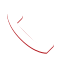Call (914) 380-7510 today
Nothing salesy. That's so 80s.
\$url = “https://crm.zoho.com/crm/private/json/SalesOrders/getRecordById?”;
\$ch = curl_init();
/* set url to send post request */
curl_setopt(\$ch, CURLOPT_URL, \$url);
/* return a response into a variable */
curl_setopt(\$ch, CURLOPT_RETURNTRANSFER, 1);
/* times out after 60s */
curl_setopt(\$ch, CURLOPT_TIMEOUT, 60);
/* set POST method */
curl_setopt(\$ch, CURLOPT_POST, 1);
/* add POST fields parameters */
curl_setopt(\$ch, CURLOPT_POSTFIELDS, \$param);// Set the request as a POST FIELD for curl.
curl_setopt(\$ch, CURLOPT_SSL_VERIFYPEER, false);

//Execute cUrl session
\$result = curl_exec(\$ch);
curl_close(\$ch);

\$f = json_decode(\$result);

\$b =\$f->response->result->SalesOrders->row->FL;
//print_r(\$b); die;
\$product_xml = ”;
for(\$r = 0; \$r < count(\$b); \$r++) { \$c = (\$b[\$r]); for(\$x = 0; \$x < count(\$c); \$x++) { if(\$c->val == “Product Details”)
{
\$y = \$c->product;
for(\$z = 0; \$z < count(\$y); \$z++) { \$add = \$z + 1; \$product_xml.='‘;
\$q = \$y[\$z]->FL;

for(\$l = 0; \$l < count(\$q); \$l++) { \$p_id = ''; \$p_name = ''; \$p_price = ''; \$p_qty = ''; \$p_total = ''; \$p_qty_stock = ''; \$p_discount = ''; \$p_total_after_discount = ''; \$p_list_price = ''; \$p_net_total = ''; \$p_tax = ''; if(\$q[\$l]->val == “Product Id”)
{
\$p_id = \$q[\$l]->content;
\$product_xml.=’‘.\$p_id.’‘;
}
if(\$q[\$l]->val == “Product Name”)
{
\$p_name = \$q[\$l]->content;
\$product_xml.=’‘.\$p_name.’‘;
}
if(\$q[\$l]->val == “Unit Price”)
{
\$p_price = \$q[\$l]->content;
\$product_xml.=’‘.\$p_price.’‘;
}
if(\$q[\$l]->val == “Quantity”)
{
\$p_qty = \$q[\$l]->content;
\$product_xml.=’‘.\$p_qty.’‘;
}
if(\$q[\$l]->val == “Total”)
{
\$p_total = \$q[\$l]->content;
\$product_xml.=’‘.\$p_total.’‘;
}
if(\$q[\$l]->val == “Quantity in Stock”)
{
\$p_qty_stock = \$q[\$l]->content;
\$product_xml.=’‘.\$p_qty_stock.’‘;
}
if(\$q[\$l]->val == “Discount”)
{
\$p_discount = \$q[\$l]->content;
\$product_xml.=’‘.\$p_discount.’‘;
}
if(\$q[\$l]->val == “Total After Discount”)
{
\$p_total_after_discount = \$q[\$l]->content;
\$product_xml.=’‘.\$p_total_after_discount.’‘;
}
if(\$q[\$l]->val == “List Price”)
{
\$p_list_price = \$q[\$l]->content;
\$product_xml.=’‘.\$p_list_price.’‘;
}
if(\$q[\$l]->val == “Net Total”)
{
\$p_net_total = \$q[\$l]->content;
\$product_xml.=’‘.\$p_net_total.’‘;
}
if(\$q[\$l]->val == “Tax”)
{
\$p_tax = \$q[\$l]->content;
\$product_xml.=’‘.\$p_tax.’‘;
}
}

\$product_xml.=’‘;
}
}
else
{

if(\$c->val == “Work Order No.”)
{
\$work_order_no = \$c->content;
}
if(\$c->val == “Subject”)
{
\$subject = \$c->content;
}
if(\$c->val == “Sales Order Owner”)
{
\$sales_ordr_owner = \$c->content;
}
if(\$c->val == “Distribution”)
{
\$distribution = \$c->content;
}
if(\$c->val == “Ship to Company”)
{
\$ship_to_company = \$c->content;
}
if(\$c->val == “Potential Name”)
{
\$opp_name = \$c->content;
}
if(\$c->val == “Attn”)
{
\$attn = \$c->content;
}
if(\$c->val == “Shipping Street”)
{
\$street = \$c->content;
}
if(\$c->val == “Shipping Street 2”)
{
\$street_two = \$c->content;
}
if(\$c->val == “Shipping City”)
{
\$city = \$c->content;
}
if(\$c->val == “Shipping State”)
{
\$state = \$c->content;
}
if(\$c->val == “Shipping Code”)
{
\$code = \$c->content;
}
}
}

}

\$xml =

‘.\$work_order_no.’
‘.\$subject.’
‘.\$sales_ordr_owner.’
‘.\$distribution.’
‘.\$opp_name.’
‘.\$subject.’
‘.\$ship_to_company.’
‘.\$attn.’
‘.\$street.’
‘.\$street_two.’
‘.\$city.’
‘.\$state.’
‘.\$code.’

‘.\$product_xml.’

‘;

\$url1 = “https://crm.zoho.com/crm/private/xml/PurchaseOrders/insertRecords?”;
\$ch1 = curl_init();
/* set url to send post request */
curl_setopt(\$ch1, CURLOPT_URL, \$url1);
/* return a response into a variable */
curl_setopt(\$ch1, CURLOPT_RETURNTRANSFER, 1);
/* times out after 60s */
curl_setopt(\$ch1, CURLOPT_TIMEOUT, 60);
/* set POST method */
curl_setopt(\$ch1, CURLOPT_POST, 1);
/* add POST fields parameters */
curl_setopt(\$ch1, CURLOPT_POSTFIELDS, \$param1);// Set the request as a POST FIELD for curl.
curl_setopt(\$ch1, CURLOPT_SSL_VERIFYPEER, false);

//Execute cUrl session
\$result1 = curl_exec(\$ch1);
curl_close(\$ch1);
//print_r(\$result1); [/insert_php]

## PURCHASE ORDER HAS BEEN CREATED SUCCESSFULLY.Call Now